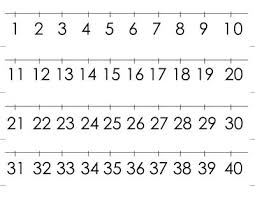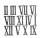# The sum 8

The sum of two numbers is 21. If three times the smaller numbers is two less than twice the larger number, find the two numbers.

a =  8
b =  13

### Step-by-step explanation:

a+b=21
3a = 2b-2

a+b=21
3•a = 2•b-2

a+b = 21
3a-2b = -2

a = 8
b = 13

Our linear equations calculator calculates it.Did you find an error or inaccuracy? Feel free to write us. Thank you!Tips to related online calculators
Do you have a system of equations and looking for calculator system of linear equations?

## Related math problems and questions:

• Two unknown numbersOne number is 2 less than a second number. Twice the second number is 14 less than 5 times the first. Find the two numbers.
• The largerThe larger of two numbers is nine more than four times the smaller number. The sum of the two numbers is fifty-nine. Find the two numbers.
• The difference 2The difference between the two numbers is 25. The smaller number is 1/6th of the larger number. What is the value of the smaller number?
• Sum of three numbersThe sum of three numbers from which the second number is 20% smaller than the first number and the third number is 25% smaller than the second number is 96. Determine these numbers.
• The sum 16The sum of A and B is 36. The difference between A & B is 8. find the larger number of A.
• Two numbersOne number is by 79 larger than the other. If we divide the larger number by the smaller one, we get the ratio 5 and the remainder 11. Determine both numbers.
• Four integersFnd four consecutive integers so that the product of the first two is 70 times smaller than the product of the next two.
• The sum 9The sum of two integers is 4. Their difference is 8. What are this two integers?
• Two-digit numberDigit sum of thinking two-digit natural number is 11. When it exchanging a sequence of digits, given a number which is 27 less than the thinking number. Find out which number I think.
• Unknown number 716% of the unknown number is by 21 less than unknown number itself. Determine the natural unknown number.
• Three figures - numbersThe sum of three numbers, if each is 10% larger than the previous one, is 662. Determine the figures.
• Two numbersThe difference between the two numbers is 74. If we divide a larger number by a smaller one, we get a quotient 7 and the rest of 2. Determine both numbers.
• Three numbersFind three numbers so that the second number is 4 times greater than the first and the third is lower by 5 than the second number. Their sum is 67.
• Two numbersThe sum of the two numbers is 1. Find both numbers if you know that half of the first is equal to one-seventh of the second number.
• NumbersDetermine the number of all positive integers less than 4183444 if each is divisible by 29, 7, 17. What is its sum?
• A number 4A number is made up of two digits. The sum of the digits is 11. if the digits are interchanged, the original number is increase by 9. Find the original number
• Two math problems1) The sum of twice a number and -6 is nine more than the opposite of that number. Find the number. 2) A collection of 27 coins, all nickels, and dimes, is worth \$2.10. How many of each coin are there? The dime, in United States usage, is a ten-cent coin.